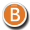• 3D Figure Assets

By:  Deva3D ()

18.50 USD• 3D Figure Assets

By:  Deva3D ()

9.25 USD
50% off• 3D Figure Assets

By:  Deva3D ()

9.25 USD
50% off• 3D Figure Assets

By:  Deva3D ()

17.00 USD• 3D Figure Assets

By:  Deva3D ()

8.25 USD
50% off• 3D Figure Assets

By:  Deva3D ()

8.25 USD
50% off• 3D Figure Assets

By:  Deva3D ()

8.50 USD
48% off• 3D Figure Assets

By:  Deva3D ()

16.50 USD• 3D Figure Assets

By:  Deva3D ()

8.50 USD
48% off• 3D Figure Assets

By:  Deva3D ()

16.50 USD• 3D Figure Assets

By:  Deva3D ()

8.50 USD
48% off• 3D Figure Assets

By:  Deva3D ()

16.50 USD• 3D Figure Assets

By:  Deva3D ()

16.49 USD• 3D Figure Assets

By:  Deva3D ()

16.49 USD• 3D Figure Assets

By:  Deva3D ()

16.49 USD• 3D Figure Assets

By:  Deva3D ()

8.50 USD
47% off• 3D Figure Assets

By:  Deva3D ()

15.95 USD• 3D Figure Assets

By:  Deva3D ()

8.50 USD
47% off• 3D Figure Assets

By:  Deva3D ()

15.90 USD• 3D Figure Assets

By:  Deva3D ()

15.90 USD• 3D Figure Assets

By:  Deva3D ()

7.95 USD
50% off• 3D Figure Assets

By:  Deva3D ()

15.90 USD• 3D Figure Assets

By:  Deva3D ()

8.50 USD
47% off• 3D Figure Assets

By:  Deva3D ()

15.90 USD• 3D Figure Assets

By:  Deva3D ()

15.50 USD• 3D Figure Assets

By:  Deva3D ()

7.75 USD
50% off• 3D Figure Assets

By:  Deva3D ()

15.50 USD• 3D Figure Assets

By:  Deva3D ()

15.50 USD• 3D Figure Assets

By:  Deva3D ()

15.50 USD• 3D Figure Assets

By:  Deva3D ()

15.50 USD• 3D Figure Assets

By:  Deva3D ()

15.50 USD• 3D Figure Assets

By:  Deva3D ()

7.50 USD
52% off• 3D Figure Assets

By:  Deva3D ()

15.50 USD• 3D Figure Assets

By:  Deva3D ()

15.50 USD• 3D Figure Assets

By:  Deva3D ()

15.50 USD• 3D Figure Assets

By:  Deva3D ()

15.50 USD• 3D Figure Assets

By:  Deva3D ()

15.50 USD• 3D Figure Assets

By:  Deva3D ()

7.50 USD
50% off• 3D Figure Assets

By:  Deva3D ()

7.99 USD
47% off• 3D Figure Assets

By:  Deva3D ()

15.00 USD• 3D Figure Assets

By:  Deva3D ()

15.00 USD• 3D Figure Assets

By:  Deva3D ()

14.90 USD• 3D Figure Assets

By:  Deva3D ()

14.90 USD• 3D Figure Assets

By:  Deva3D ()

14.90 USD• 3D Figure Assets

By:  Deva3D ()

14.90 USD• 3D Figure Assets

By:  Deva3D ()

7.50 USD
50% off• 3D Figure Assets

By:  Deva3D ()

7.50 USD
50% off• 3D Figure Assets

By:  Deva3D ()

7.90 USD
47% off• 3D Figure Assets

By:  Deva3D ()

7.99 USD
46% off• 3D Figure Assets

By:  Deva3D ()

7.99 USD
46% off• 3D Figure Assets

By:  Deva3D ()

14.90 USD• 3D Figure Assets

By:  Deva3D ()

14.50 USD• 3D Figure Assets

By:  Deva3D ()

14.50 USD• 3D Figure Assets

By:  Deva3D ()

14.50 USD